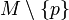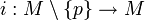# Point-deletion inclusion induces isomorphism on fundamental groups for manifold of dimension at least two

## Statement

Suppose$M$ is a connected manifold of dimension at least two. Suppose$p$ is a point in$M$. Note that the manifold$M \setminus \{ p \}$ is still a connected manifold (because$M$ has dimension at least two). Consider the inclusion map:$i: M \setminus \{ p \} \to M$

This induces a homomorphism between the Fundamental group (?)s (note that it is not necessary to specify basepoints because both manifolds are path-connected):$\pi_1(i): \pi_1(M \setminus \{ p \}) \to \pi_1(M)$

This induced map is an isomorphism. In particular, both the fundamental groups are isomorphic groups.

## Facts used

1. Seifert-van Kampen theorem